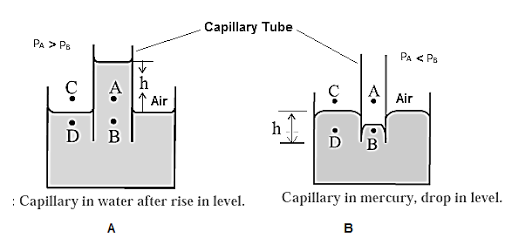# Explain the capillary action

Explain the capillary action

verified

When a capillary tube is partially immersed in a wetting liquid, there is a capillary rise and the liquid meniscus inside the tube is concave, as shown in Figure (a).
Consider four points A, B, C, D, of which point A is just above the concave meniscus inside the capillary, and point B is just below it. Points C and D are just above and below the free liquid surface outside.
Let PA, PB, PC, and PD be the pressures at points A, B, C, and D respectively.

Now, PA = PC = atmospheric pressure

The pressure is the same on both sides of the free surface of a liquid, so thatExplanation of (a) capillary rise
(b) capillary depression

PC = PD

∴ PA = PD

The pressure on the concave side of a meniscus is always greater than that on the convex side, so that

PA > PB

∴ PD > PB  ........(∵ PA = PD)

The excess pressure outside presses the liquid up the capillary until the pressures at B and D (at the same horizontal level) equalize, i.e., PB becomes equal to PD. Thus, there is a capillary rise.

For a non-wetting liquid, there is capillary depression and the liquid meniscus in the capillary tube is convex, as shown in Figure (b).

Consider again four points A, B, C, and D when the meniscus in the capillary tube is at the same level as the free surface of the liquid. Points A and B are just above and below the convex meniscus. Points C and D are just above and below the free liquid surface outside.

The pressure at B(PB) is greater than that at A(PA). The pressure at A is the atmospheric pressure H and at D, PD ≃ H = PA. Hence, the hydrostatic pressure at the same levels at B and D are not equal, PB >PD. Hence, the liquid flows from B to D, and the level of the liquid in the capillary falls. This continues till the pressure at B' is the same as that D', that is till the pressures at the same level are equal